# Kepler's Laws

Johannes Kepler, working with data painstakingly collected by Tycho Brahe without the aid of a telescope, developed three laws which described the motion of the planets across the sky.

1. The Law of Orbits: All planets move in elliptical orbits, with the sun at one focus.

2. The Law of Areas: A line that connects a planet to the sun sweeps out equal areas in equal times.

3. The Law of Periods: The square of the period of any planet is proportional to the cube of the semimajor axis of its orbit.

Kepler's laws were derived for orbits around the sun, but they apply to satellite orbits as well.

Index

Gravity concepts

Orbit concepts

 HyperPhysics***** Mechanics R Nave
Go Back

# The Law of Orbits

## All planets move in elliptical orbits, with the sun at one focus.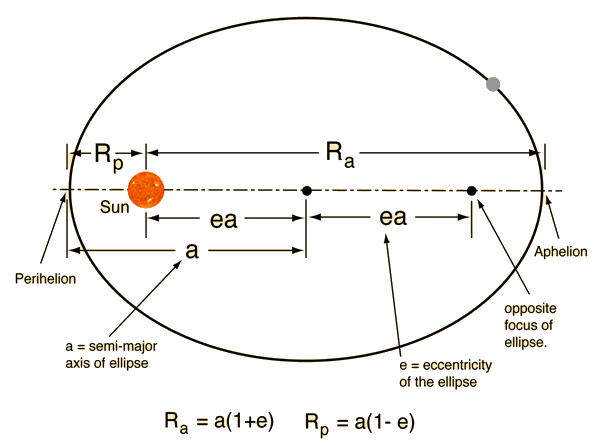This is one of Kepler's laws. The elliptical shape of the orbit is a result of the inverse square force of gravity. The eccentricity of the ellipse is greatly exaggerated here.

 Describing an ellipse
 Developing Kepler's Law of Orbits
Index

Gravity concepts

Orbit concepts

 HyperPhysics***** Mechanics R Nave
Go Back# Orbit Eccentricity

The eccentricity of an ellipse can be defined as the ratio of the distance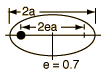between the foci to the major axis of the ellipse. The eccentricity is zero for a circle. Of the planetary orbits, only Pluto has a large eccentricity.
 Eccentricity examples

Index

Gravity concepts

Orbit concepts

 HyperPhysics***** Mechanics R Nave
Go Back

# Examples of Ellipse Eccentricity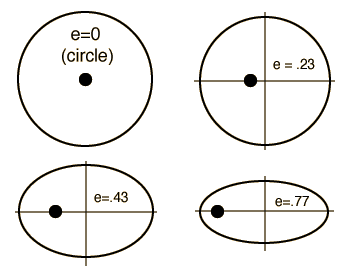Planetary orbit eccentricities
 Mercury 0.206 Venus 0.0068 Earth 0.0167 Mars 0.0934 Jupiter 0.0485 Saturn 0.0556 Uranus 0.0472 Neptune 0.0086 Pluto 0.25

 Outer planets

Index

Gravity concepts

Orbit concepts

 HyperPhysics***** Mechanics R Nave
Go Back

# The Law of Areas

## A line that connects a planet to the sun sweeps out equal areas in equal times.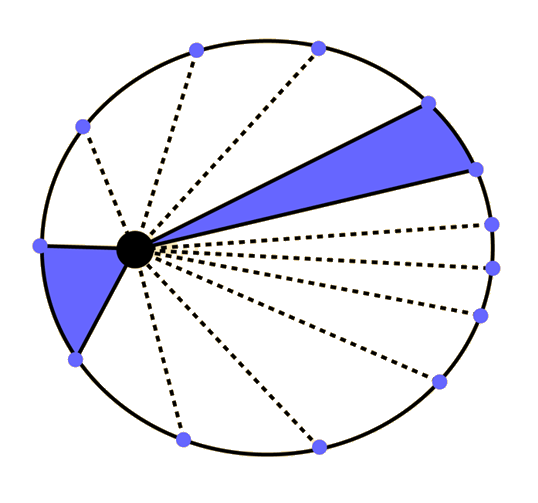This is one of Kepler's laws.This empirical law discovered by Kepler arises from conservation of angular momentum. When the planet is closer to the sun, it moves faster, sweeping through a longer path in a given time.
 Development of the Law of Areas
Index

Gravity concepts

Orbit concepts

 HyperPhysics***** Mechanics R Nave
Go Back

# The Law of Periods

## The square of the period of any planet is proportional to the cube of the semimajor axis of its orbit.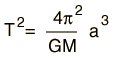This is one of Kepler's laws.This law arises from the law of gravitation. Newton first formulated the law of gravitation from Kepler's 3rd law.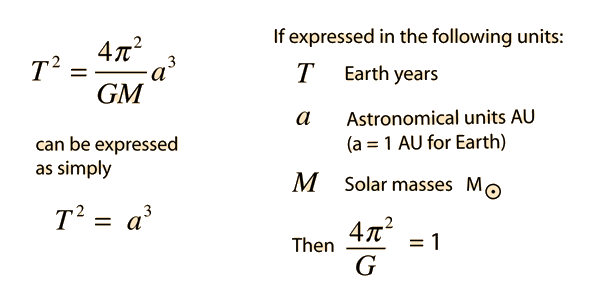Kepler's Law of Periods in the above form is an approximation that serves well for the orbits of the planets because the Sun's mass is so dominant. But more precisely the law should be writtenIn this more rigorous form it is useful for calculation of the orbital period of moons or other binary orbits like those of binary stars.

 Table of data
 Development of the Law of Periods
Index

Gravity concepts

Orbit concepts

 HyperPhysics***** Mechanics R Nave
Go Back

# Data: Law of Periods

Data confirming Kepler's Law of Periods comes from measurements of the motion of the planets.

 Planet Semimajor axis (1010m) PeriodT (y) T2/a3(10-34y2/m3) Mercury 5.79 0.241 2.99 Venus 10.8 0.615 3.00 Earth 15.0 1 2.96 Mars 22.8 1.88 2.98 Jupiter 77.8 11.9 3.01 Saturn 143 29.5 2.98 Uranus 287 84 2.98 Neptune 450 165 2.99 Pluto 590 248 2.99

The quantity T2/a3 depends upon the sum of the masses of the Sun and the planet, but since the mass of the Sun is so great, adding the mass of the planet makes very little difference.

Data from Halliday, Resnick, Walker, Fundamentals of Physics 4th Ed Extended. Table 15-3

Index

Gravity concepts

Orbit concepts

 HyperPhysics***** Mechanics R Nave
Go Back